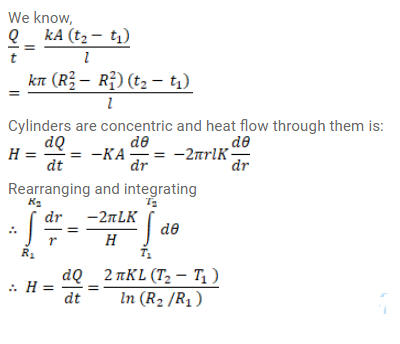# A hollow tube has a length L,Question:

A hollow tube has a length $L$, inner radius $R_{1}$ and the outer radius $R_{2}$. The material has a thermal conductivity $\mathrm{K}$. Find the heat flowing through the walls of the tube if

(a) the flat ends are maintained at temperature $T_{1}$ and $T_{2}\left(T_{1}>T_{2}\right)$ and the inside of the tube is maintained at temperature $T_{1}$ and the outside is maintained at $\mathrm{T}_{2}$.

Solution: